# 参考文献

GB/T 29171-2012 岩石毛管压力曲线的测定
SY/T 6738-2008 岩心毛管压力测量仪器通用技术条件
《岩石物理学》第三版[美] Djebbar Tiab，[美] Erle C.Donaldson 著，周灿灿，胡法龙，李潮流 等 译

# 毛管压力

$$p_c = p_{nw} - p_w$$

$p_{nw}$：非润湿相流体压力；
$p_w$：润湿相流体压力，通常为水相。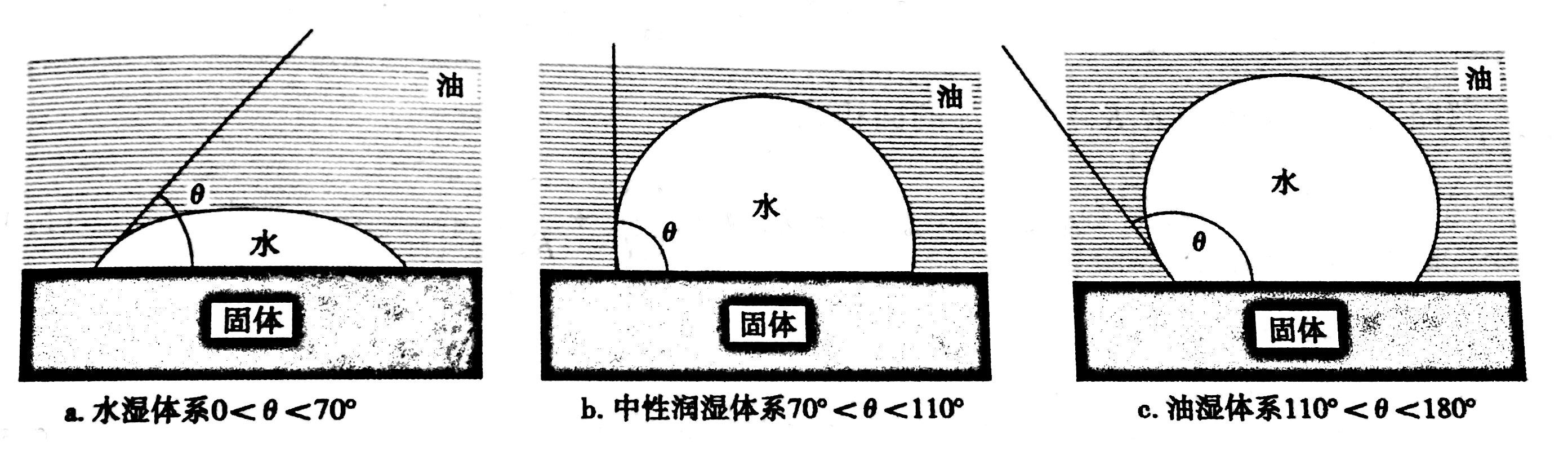a:非混相流体接触。水润湿固体表面；油是非润湿相且滞留在润湿相流体界面膜上，非润湿相中压力>润湿相，结果流体界面呈凸型。
b:油水对固体表面的润湿程度相等，每相流体中压力相等。两相流体间界面垂直于固体表面而且毛管压力为0。
c:水相压力大于油相压力时，界面曲率指向油相且毛管压力为正。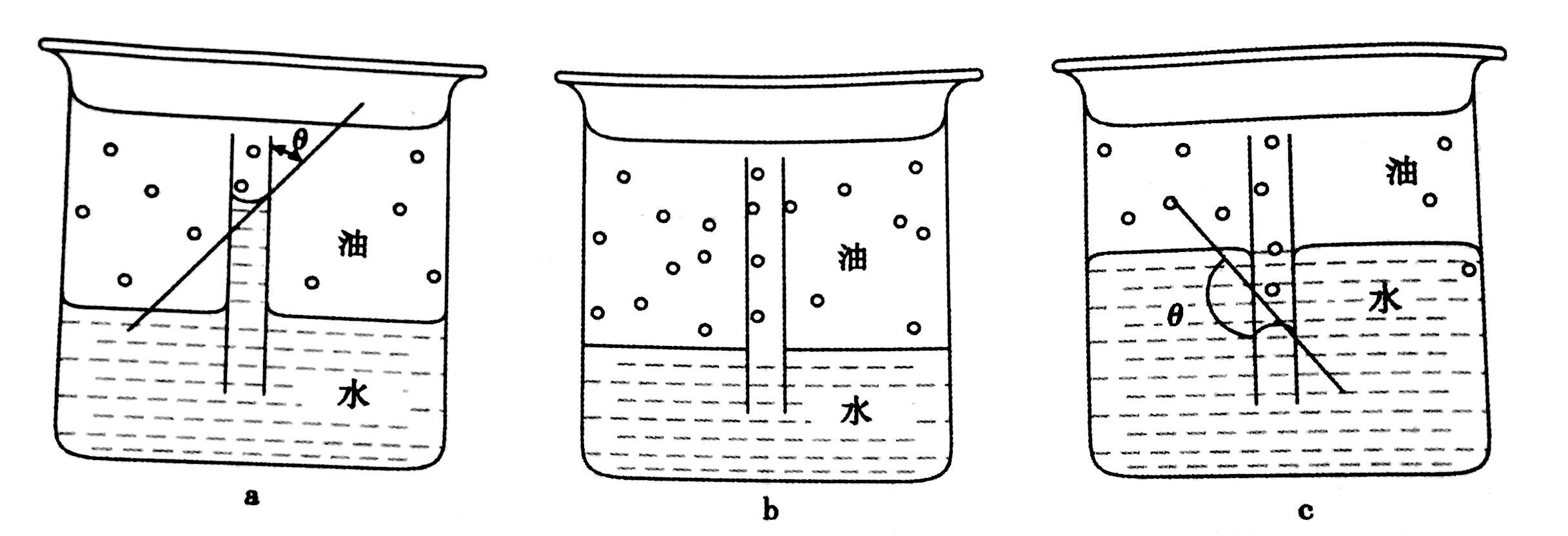# 压汞法

## 原理

$$P_c = \frac{2\delta cos\theta}{r_c}$$

$P_c$：毛管压力（绝对压力）的数值，MPa;
$\delta$：表面张力的数值，N/m；
$\theta$：润湿接触角，°；
$r_c$：毛管半径数值，μm。

$$P_c = \frac{0.735}{r_c}$$

## 用途

1.进退汞曲线
2.最大进汞饱和度——求取束缚水饱和度
3.孔候半径分布频率
4.排驱压力——最大毛管半径
5.饱和度中值压力——中值半径

# 离心机法

## 原理

$$p_{ci} = 1.097 × 10^{-9} \Delta\rho L (R_e - \frac{L}{2}) n^2$$

$p_{ci}$：岩样驱替毛管压力的数值，MPa；
$\Delta\rho$：两相流体密度差的数值，g/cm^3；
$L$：岩样长度，cm；
$R_e$：岩样的外旋转半径，cm；
$n$：离心机转速，r/min；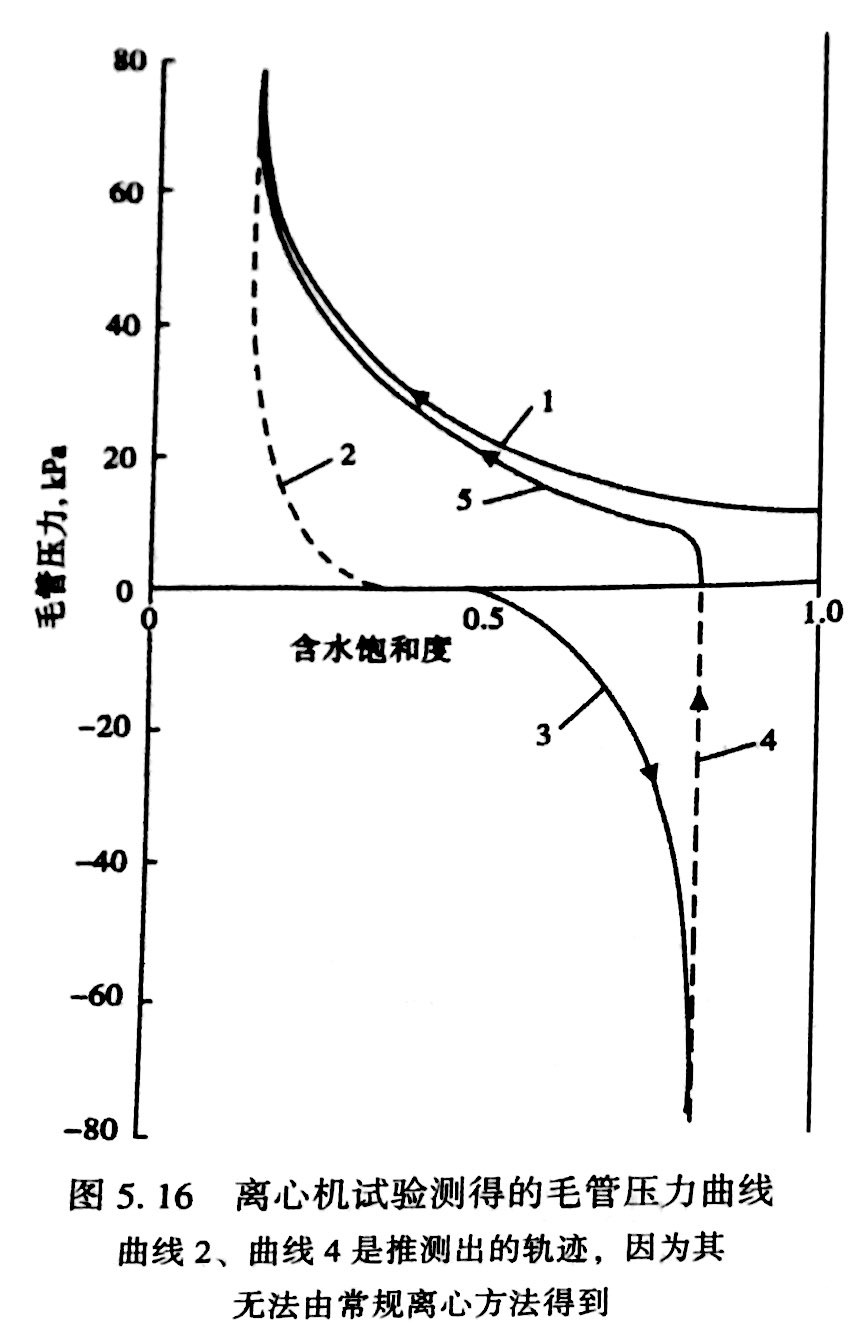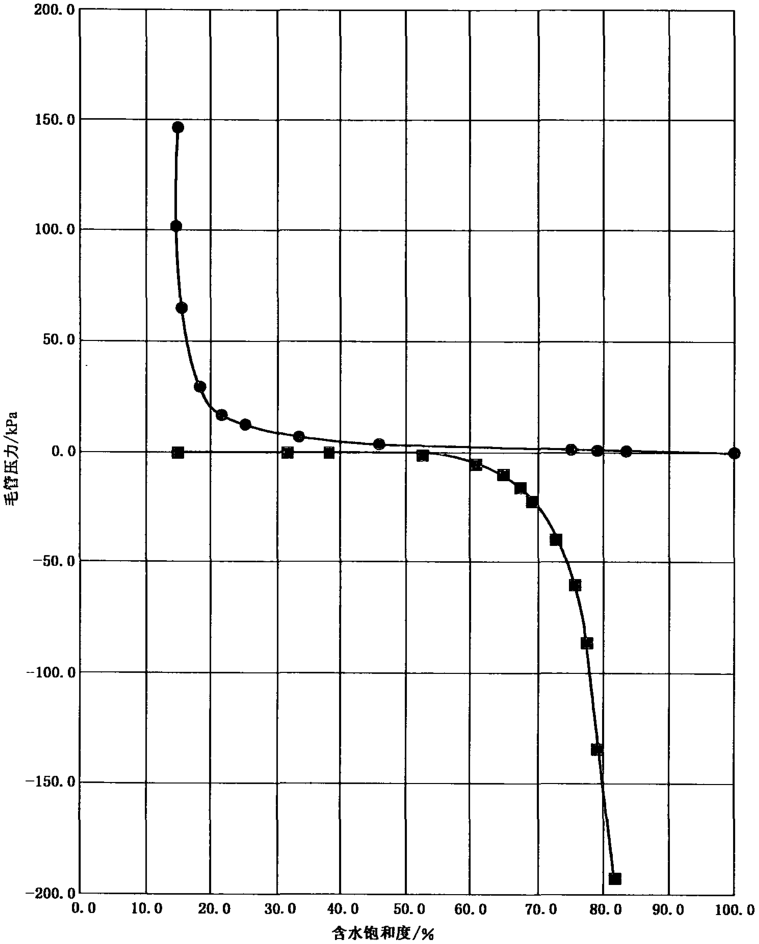## 仪器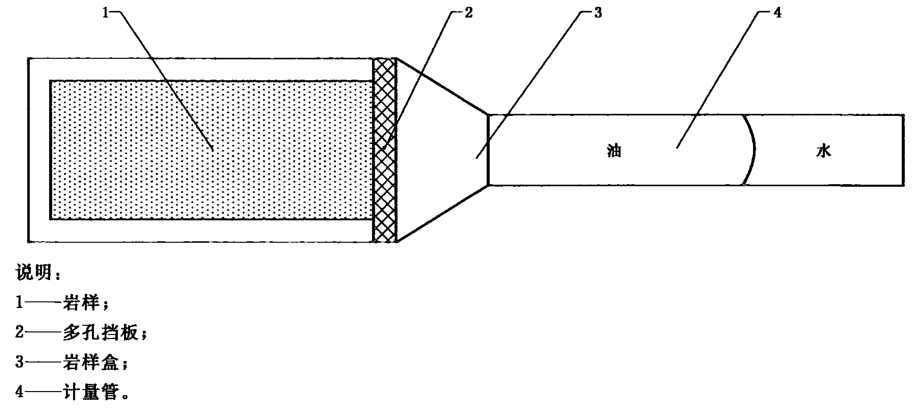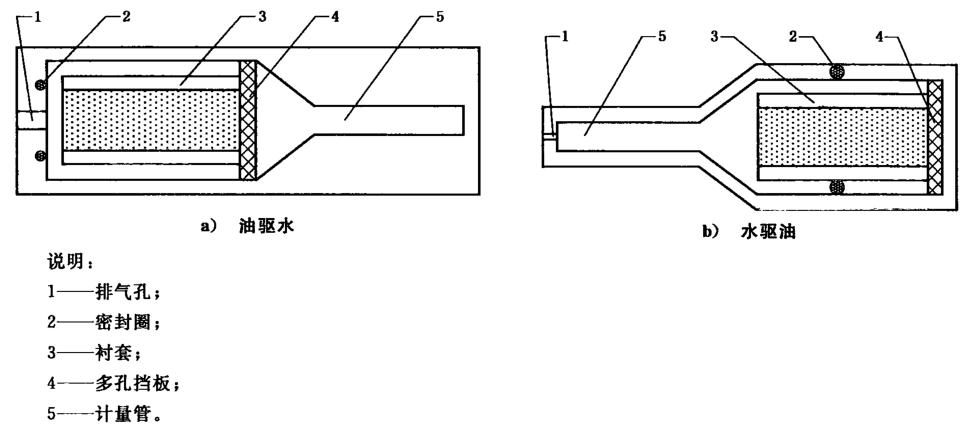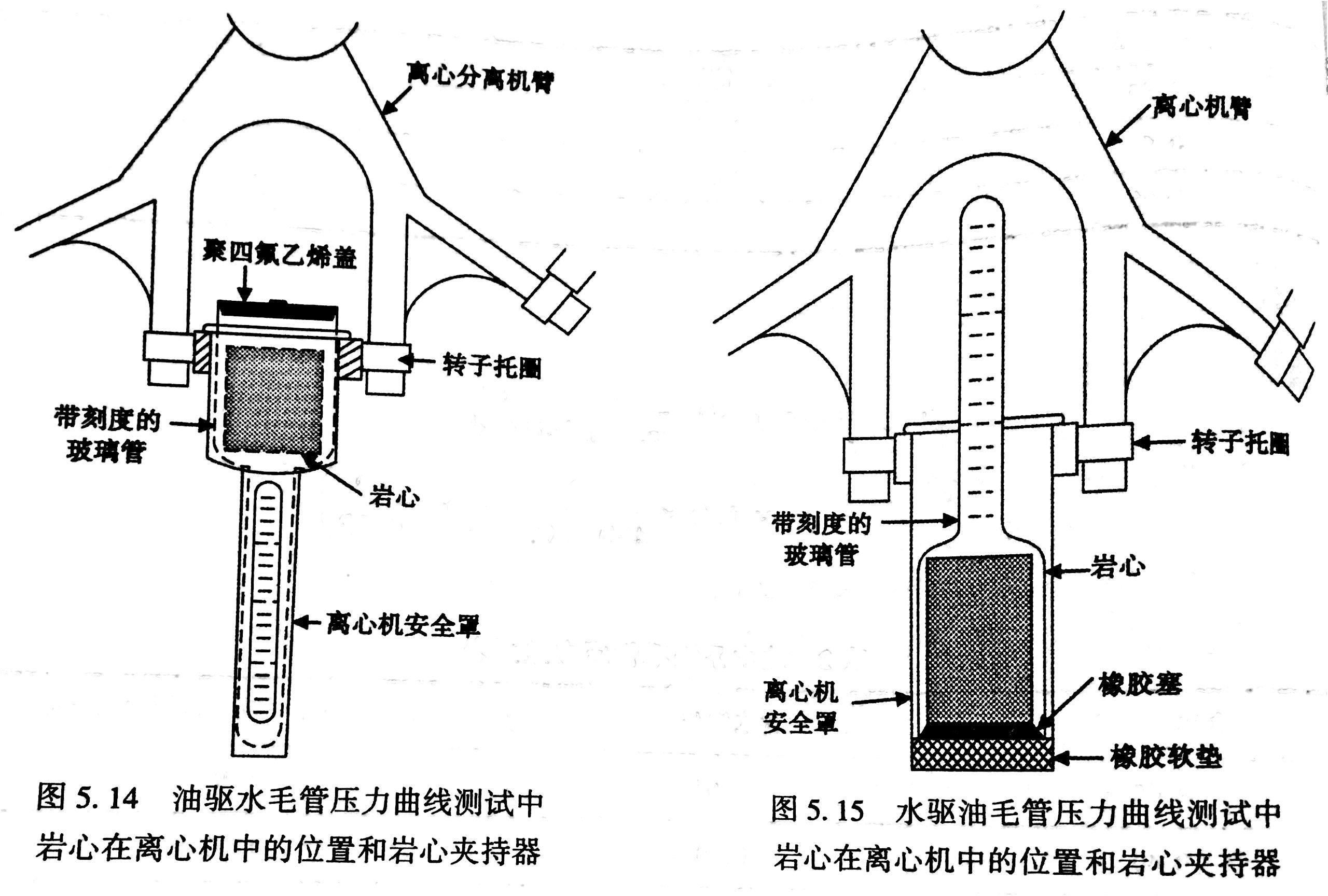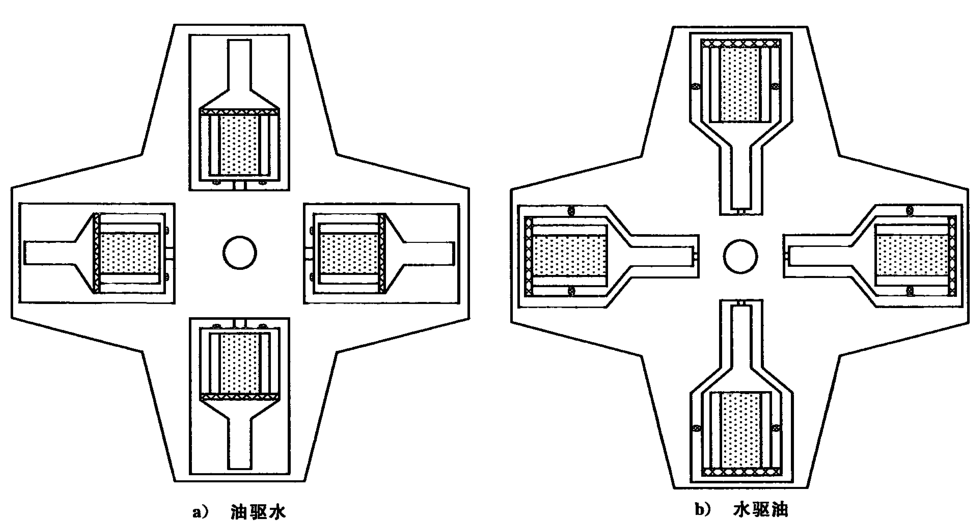1.孔隙度
2.孔喉半径
3.残余油

# 半渗透隔板

## 原理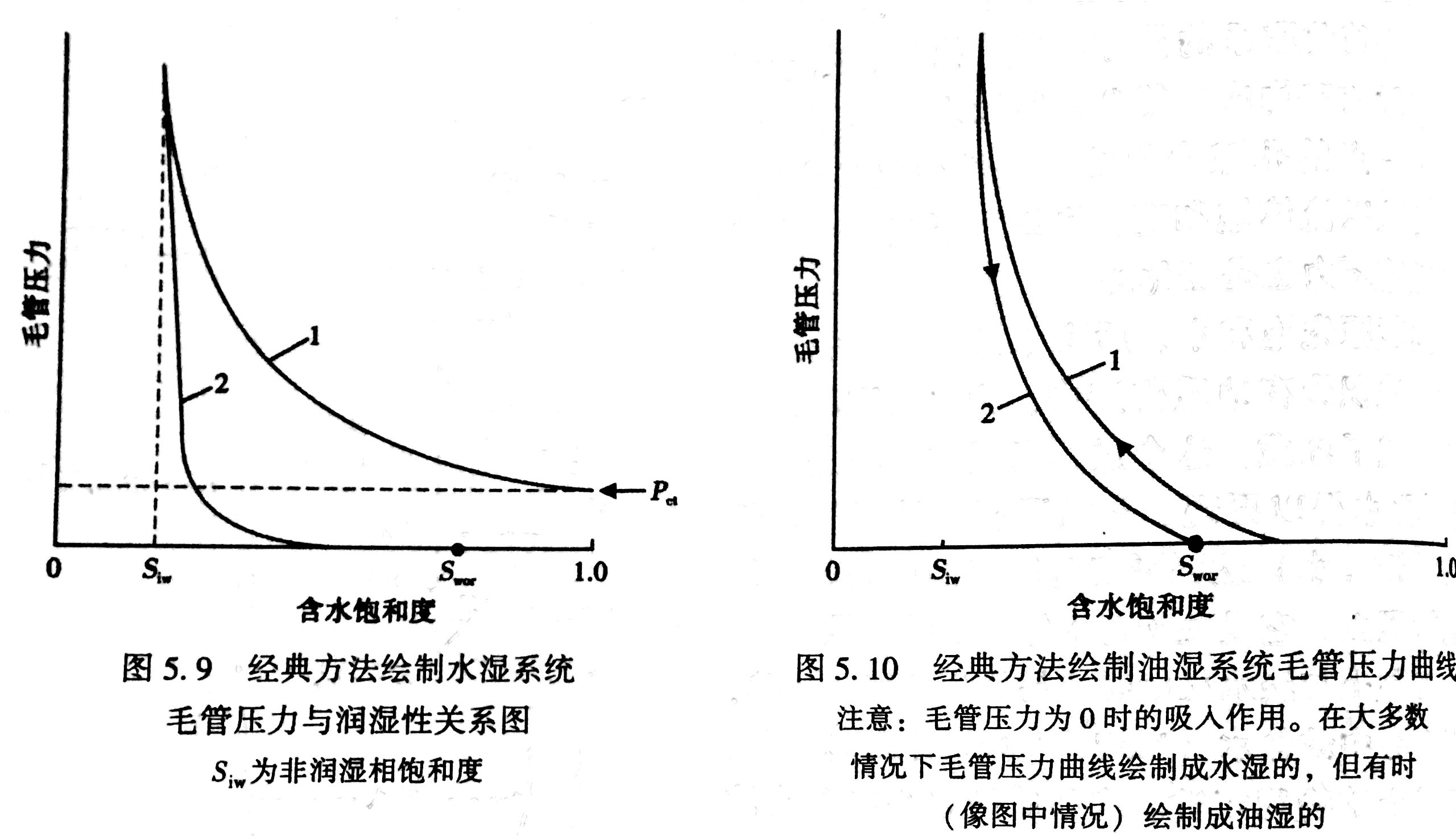## 仪器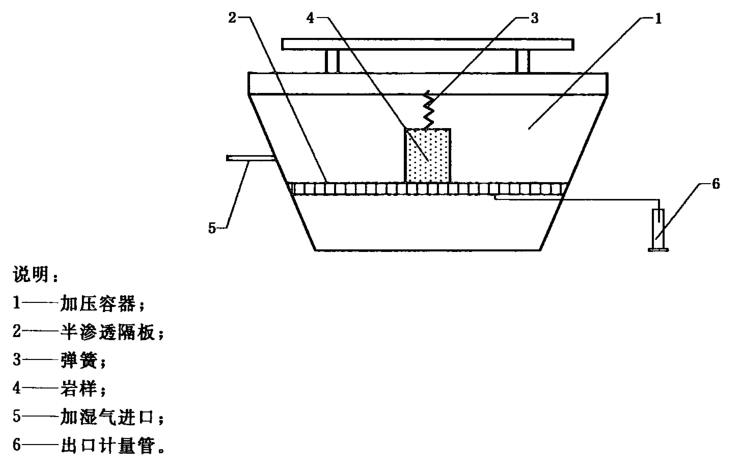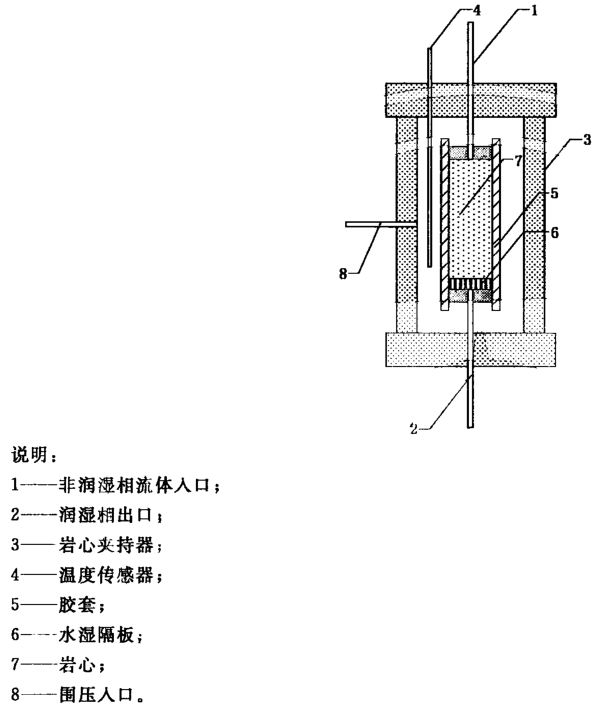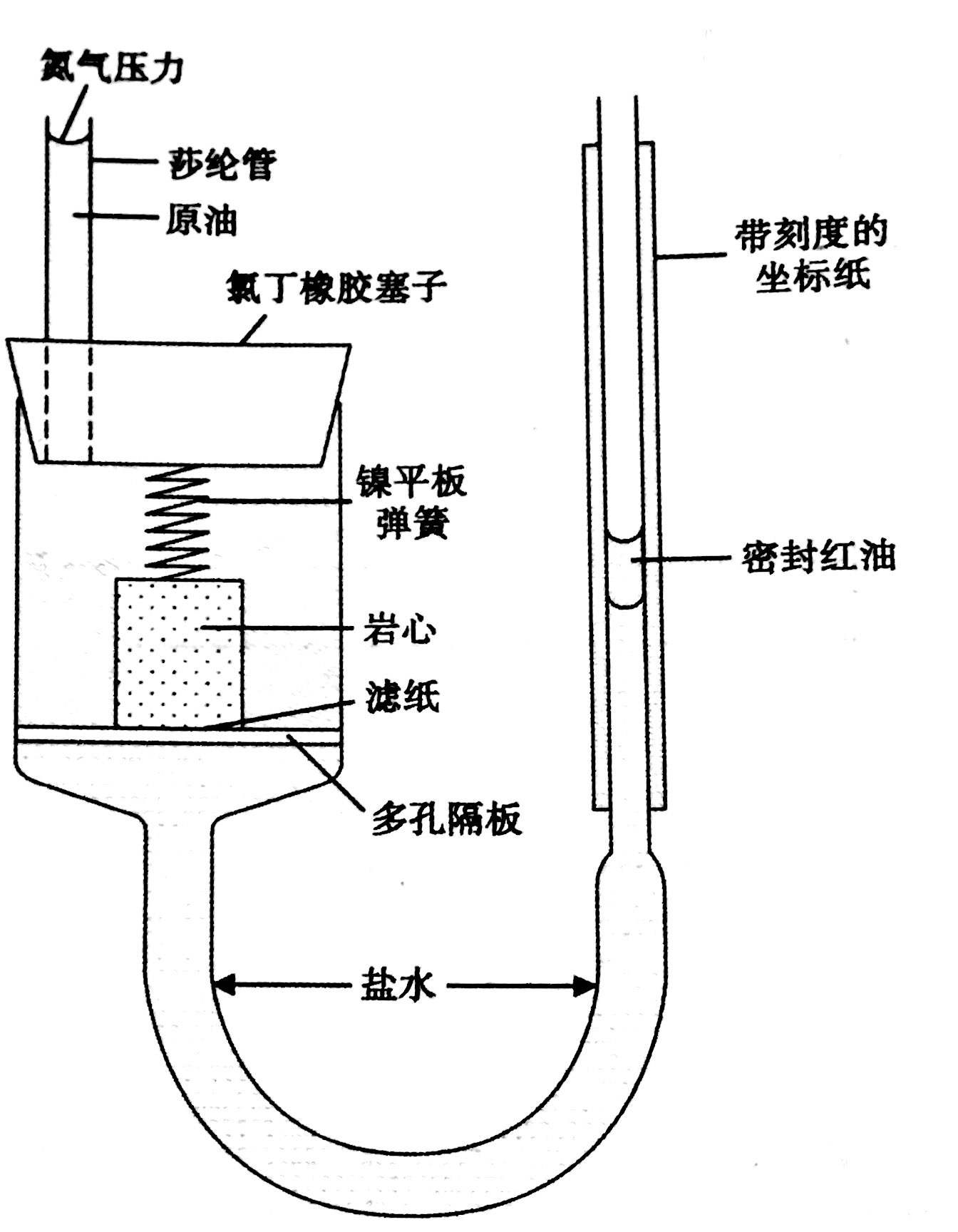1.确定束缚水饱和度
2.门限压力
3.孔喉半径